Refer to our Texas Go Math Grade 4 Answer Key Pdf to score good marks in the exams. Test yourself by practicing the problems from Texas Go Math Grade 4 Unit 1 Assessment Answer Key.

Vocabulary
unit fraction
benchmark
decimal

Choose the best term from the box.

Question 1.
A ____________ is a known size or amount that helps you understand another size or amount.
Explanation:
A benchmark is a known size or amount that helps you understand a different size or amount. You can use 1/2 as a benchmark to help you compare fractions.

Question 2.
A ____________ always has a numerator of 1.
Explanation:
a unit fraction can be defined as a fraction whose numerator is 1. It represents 1 shaded part of all the equal parts of the whole.

Concepts and Skills

Write the decimal shown by the model. The flat represents 1 unit.

Question 3.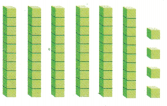Explanation:
A long represents 10 cubes
and the small cube is 1

Question 4.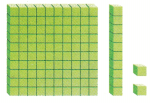Explanation:
Flat is represented as 1 unit.
A long represents 10 cubes
and the small cube is 1

Compare. Write <, >, or =. Shade the model to help.

Question 5.
0.45 _______ 0.84Explanation:
0.45 is lesser than 0.84

Question 6.
0.74 _______ 0.74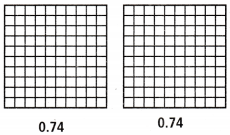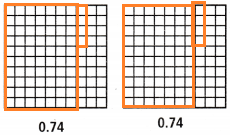Explanation:
0.74 = 0.74

Write the fraction in simplest form.

Question 7.
$$\frac{6}{9}$$
$$\frac{2}{3}$$
Explanation:
3 x 2 = 6
3 x 3 = 9
Written the simplest form of the fraction.

Question 8.
$$\frac{4}{10}$$
$$\frac{2}{5}$$
Explanation:
2 x 2 = 4
2 x 5 = 10
Written the simplest form of the fraction.

Question 9.
$$\frac{8}{12}$$
$$\frac{4}{6}$$
Explanation:
2 x 4 = 8
2 x 6 = 12
Written the simplest form of the fraction.

Question 10.
$$\frac{3}{15}$$
$$\frac{1}{5}$$
Explanation:
3 x 1= 3
3 x 5= 15
Written the simplest form of the fraction.

Use the properties and mental math to find the sum.

Question 11.
$$\left(\frac{3}{10}+\frac{4}{10}\right)+\frac{6}{10}$$
$$\left(\frac{13}{10}] Explanation: [latex]\frac{3}{10}$$ + $$\frac{4}{10}$$ + $$\frac{6}{10}$$
Denominators are same
$$\frac{6 + 3 + 4}{10}$$
$$\frac{13}{10}$$
Written the simplest form of the fraction.

Question 12.
$$2 \frac{4}{6}+\left(2 \frac{1}{6}+2 \frac{2}{6}\right)$$
$$\frac{43}{6}$$

Explanation:
$$\frac{16}{6}$$ + $$\frac{13}{6}$$ + $$\frac{14}{6}$$
Denominators are same
$$\frac{16 + 13 + 14}{6}$$
$$\frac{43}{6}$$
Written the simplest form of the fraction.

Question 13.
$$\frac{3}{12}+\left(2 \frac{9}{12}+\frac{6}{12}\right)$$
$$\frac{39}{12}$$
Explanation:
$$\frac{3}{12}$$ + $$\frac{33}{12}$$ + $$\frac{6}{12}$$
Denominators are same
$$\frac{3 +33 + 6}{12}$$
$$\frac{39}{12}$$
Written the simplest form of the fraction.

Question 14.
Pam paid for her lunch with the amount of money shown below.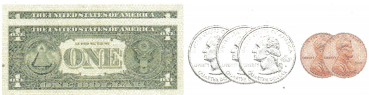(A) 2.73; 2$$\frac{73}{100}$$
(B) 2.78; 2$$\frac{78}{100}$$
(C) 2.7; 2$$\frac{7}{100}$$
(D) 2.77; 2$$\frac{73}{100}$$

Question 15.
Miguel’s class went to the state fair. The fairground is divided into sections. Rides are in $$\frac{6}{10}$$ of the fairground. Games are in $$\frac{2}{10}$$ of the fairground. Farm exhibits are in $$\frac{1}{10}$$ of the fairground. Which fraction of the fairgrounds has rides, games, and farm exhibits?
(A) $$\frac{3}{10}$$
(B) $$\frac{9}{10}$$
(C) $$\frac{8}{10}$$
(D) $$\frac{4}{10}$$
Explanation:
$$\frac{6}{10}$$+ $$\frac{2}{10}$$ + $$\frac{1}{10}$$
Denominators are same for all
$$\frac{6 + 2 + 1}{10}$$
$$\frac{9}{10}$$

Question 16.
In Kelly’s class, $$\frac{1}{4}$$ of the students said that blue was their favorite color. Another $$\frac{1}{4}$$ of the students said yellow was their favorite color. Kelly added the fractions and found the sum was $$\frac{3}{8}$$. Which statement best describes the sum $$\frac{3}{8}$$?
(A) It is reasonable because $$\frac{1}{2}$$ + 0 = $$\frac{1}{2}$$.
(B) It is reasonable because $$\frac{1}{4}$$ + $$\frac{1}{2}$$ = 1.
(C) It is not reasonable because $$\frac{1}{4}$$ + $$\frac{1}{4}$$ = $$\frac{1}{2}$$.
(D) It is not reasonable because $$\frac{1}{2}$$ + $$\frac{1}{2}$$ = 1.
Explanation:
it is not reasonable because $$\frac{1}{4}$$ + $$\frac{1}{4}$$ = $$\frac{1}{2}$$.

Question 17.
Pike National Forest located in California has a total area of 871,495 acres. What is the area rounded to the nearest thousand?
(A) 800,000
(B) 870,00
(C) 871,000
(D) 900,000
Explanation:
871,000 is the area rounded to the nearest thousand

Question 18.
Carson shaded a model to represent the parts of the books he read this weekend. Which decimal written in standard and expanded form represents the parts of the books he read?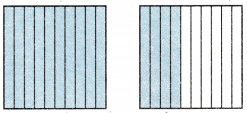(A) 4.4;4 + 0.4
(B) 0.44; 0.4 + 0.04
(C) 1.4; 1 + 0.4
(D) 1.14; 1 + 0.1 + 0.04
Explanation:
1.4; 1 + 0.4 is the  decimal written in standard and expanded form represents the parts of the books he read

Question 19.
Pablo is training for a marathon. He ran 5$$\frac{4}{8}$$ mites on Friday, 6$$\frac{1}{8}$$ miles on Saturday, and 7$$\frac{2}{8}$$ mites on Sunday. How many miles did he run on all three days?
(A) 1$$\frac{5}{8}$$ miles
(B) 12$$\frac{1}{8}$$ miles
(C) 18$$\frac{4}{8}$$ miles
(D) 18$$\frac{7}{8}$$ miles
Explanation:
5$$\frac{4}{8}$$ + 6$$\frac{1}{8}$$ + 7$$\frac{2}{8}$$
$$\frac{44}{8}$$ + $$\frac{49}{8}$$+$$\frac{58}{8}$$
18$$\frac{7}{8}$$ miles he run on all three days

Question 20.
The total attendance for all baseball games for 2011 was 78,588,004. Which number below is greater than 78,588,004?
(A) 75,858,004
(B) 78,585,041
(C) 78,588,014
(D) 78,587,001
Explanation:
78,588,014 is greater than 78,588,004

Question 21.
Erica’s high score on her new video game is 31,000 points. Maria’s high score is $$\frac{1}{10}$$ of Erica’s. How do you write Maria’s score in expanded form?
(A) 30 + 1
(B) 3,000 + 100
(C) 300 + 1
(D) 30,000 + 1,000
Explanation:
Maria’s score in expanded form is 30,000 + 1,000

Question 22.
Lisa mixed 4$$\frac{4}{6}$$ cups of orange juice with 3$$\frac{1}{6}$$ cups of milk to make a health shake. She drank 3$$\frac{3}{6}$$ cups of the health shake. How much of the health shake did Lisa not drink?
(A) $$\frac{2}{3}$$
(B) 4$$\frac{2}{6}$$
(C) 7$$\frac{5}{6}$$
(D) 11$$\frac{2}{6}$$
Explanation:
Lisa not drink 4$$\frac{2}{6}$$ of the health shake

Question 23.
At Mika’s party, there was 1 pepperoni pizza. The pizza was cut into 8 slices, Two people each ate a slice of pizza. Mika subtracted to find out how much pizza was left. Which statement best describes the difference $$\frac{6}{8}$$?
(A) It is reasonable because 1 – $$\frac{3}{4}$$ = $$\frac{1}{4}$$
(B) It is reasonable because 1 – $$\frac{1}{4}$$ = $$\frac{3}{4}$$
(C) It is not reasonable because $$\frac{3}{4}$$ – $$\frac{1}{4}$$ = $$\frac{1}{2}$$.
(D) It is not reasonable because $$\frac{1}{4}$$ + $$\frac{1}{4}$$ = $$\frac{1}{2}$$.
Explanation:
It is reasonable because 1 – $$\frac{1}{4}$$ = $$\frac{3}{4}$$ is the statement best describes the difference $$\frac{6}{8}$$

Question 24.
Ann needs $$\frac{2}{8}$$ gallon of fruit juice and $$\frac{4}{8}$$ gallon of orange juice to make punch. She needs an amount of sparkling water that is equal to the amount of fruit juice and orange juice combined. In simplest form, how much sparkling water does she need?
(A) $$\frac{2}{8}$$gallon
(B) $$\frac{3}{4}$$gallon
(C) $$\frac{1}{2}$$gallon
(D) Not here
she need $$\frac{3}{4}$$gallon  of sparkling water